Courses

# Electromagnetic Fields 3 MCQ

## 20 Questions MCQ Test Mock Test Series for SSC JE Electrical Engineering | Electromagnetic Fields 3 MCQ

Description
This mock test of Electromagnetic Fields 3 MCQ for Electrical Engineering (EE) helps you for every Electrical Engineering (EE) entrance exam. This contains 20 Multiple Choice Questions for Electrical Engineering (EE) Electromagnetic Fields 3 MCQ (mcq) to study with solutions a complete question bank. The solved questions answers in this Electromagnetic Fields 3 MCQ quiz give you a good mix of easy questions and tough questions. Electrical Engineering (EE) students definitely take this Electromagnetic Fields 3 MCQ exercise for a better result in the exam. You can find other Electromagnetic Fields 3 MCQ extra questions, long questions & short questions for Electrical Engineering (EE) on EduRev as well by searching above.
QUESTION: 1

### The classical analogous of a simple lever is______;

Solution:

A lever maintains the same level of work required to lift something as if it weren't used.

W = Fd

The amount of force required is decreased, but the distance that you need to move is increased while the work remains constant.

The amount of Power going into a transformer is the same amount that is coming out (not accounting for losses)

Power = Voltage × Current

P = VI

You can increase the voltage of a transformer, but the current will vary inversely as the power remains unchanged.

QUESTION: 2

### What is the relation between magnetic field intensity H and magnetic flux density?

Solution:

The relation between magnetic field intensity (H) and magnetic flux density (B) is

B = μ H Wb/m2

Where, μ is the magnetic permeability

QUESTION: 3

### A 1 ϕ, 120 V, 60 Hz supply is connected to the coil as shown below. The coil has 200 turns. What is the maximum flux developed by the coil?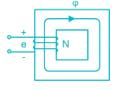Solution:

Given that, E = 120 V

Number of turns (N) = 200

We know that,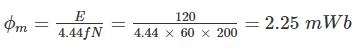QUESTION: 4

The magnetic field intensity in a material whose relative permeability is 1 when the flux density is 0.005 T is:

Solution:

The relation between magnetic field intensity (H) and magnetic flux density (B) is

B = μ H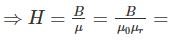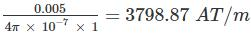QUESTION: 5

Curie point is:

Solution:

The Curie temperature or Curie point, is the temperature above which certain materials lose their permanent magnetic properties, to be replaced by induced magnetism.

QUESTION: 6

Two magnetic cores A and B made up of materials with relative permeability 300 and 400 respectively have identical physical dimension then_______.

Solution:

The reluctance is inversely proportional to the relative permeability. Hence material with lesser permeability will offer more reluctance.

Here core A has lesser permeability and hence offer more reluctance.

QUESTION: 7

Paramagnetic substances are:

Solution:

Those substances which are weekly magnetised when placed in an external magnetic field in the same direction as the applied field are called paramagnetic substances. They tend to move from weaker to the stronger part of the field. The magnetism exhibited by these substances is called Para magnetism.

Examples: Aluminium, platinum, sodium, calcium

QUESTION: 8

What is the energy stored in the magnetic field at a solenoid of 40m long and 4m diameter wound with 100 turns of wire carrying a current at 20 A?

Solution:

The inductance of a solenoid is given by,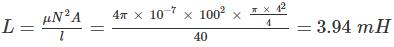Energy stored in an inductor is given by,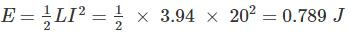QUESTION: 9

Material subjected to rapid reversal of magnetism should have:

Solution:

Permeability is the measure of the ability of a material to support the formation of a magnetic field within itself. It is the degree of magnetization that a material obtains in response to an applied magnetic field. Hence permeability should be high to rapid reversal of magnetism.

Hysteresis loss is caused by the magnetization and demagnetization of the core as current flows in the forward and reverse directions. Hence it should be low.

QUESTION: 10

Calculate the reluctance of a magnetic coil which is wounded uniformly on an iron core provided that the relative permeability of the iron is 1400. Also, the length of the magnetic circuit is 70 cm and the cross-sectional area of the core is 5 cm2.

Solution:

Reluctance of a magnetic coil is given by,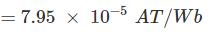QUESTION: 11

The unit of Magmetomotive Foce (MMF) is

Solution:

The current flowing in an electric circuit is due to the existence of electromotive force similarly magnetomotive force (MMF) is required to drive the magnetic flux in the magnetic circuit. The magnetic pressure, which sets up the magnetic flux in a magnetic circuit is called Magnetomotive Force. The SI unit of MMF is ampere-turn (AT)

QUESTION: 12

Flux in a magnetic circuit is analogous to:

Solution: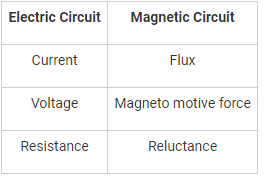QUESTION: 13

Find the magnetic field strength if a coil of 180 turns is wound uniformly over a wooden ring having a mean circumferences of 0.5 m and with current of 4A through the coil

Solution:

Given that, Number of turns (N) = 180

Length (l) = 0.5 m

Current (I) = 4 A

Magnetic field strength (H) is given by,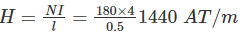QUESTION: 14

Temporary magnets are used in

Solution:

Temporary magnets are made from soft metals and only retain their magnetism while near a permanent magnetic field or electronic current. Common temporary magnets include nails and paperclips, which can be picked up or moved by a strong magnet

Temporary magnets are used in telephones, loud speakers, generators and electric motors

QUESTION: 15

The magnetism left in the iron after exciting field has been removed is known as

Solution:

The ability of a coil to retain some of its magnetism within the core after the magnetisation process has stopped is called retentivity, while the amount of flux density still remaining in the core is called residual magnetism

QUESTION: 16

The magnetic field strength on the axis of a short solenoid is:

Solution:

The magnetic field strength (H) of a short solenoid is given by,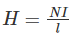Where N is the number turns

I is the current in ampere

L is the length

From the above expression we can observe that the magnetic field strength is proportional to ampere turns and inversely proportional to length.

QUESTION: 17

The electric field intensity due to an infinite plane sheet of charge is

Solution:

The electric field intensity due to an infinite plane sheet of charge is given by,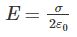Thus, the field is uniform and does not depend on the distance from the plane sheet of charge.

QUESTION: 18

What is the unit of reciprocal of reluctance?

Solution:

Permeance is the reciprocal of reluctance. In a magnetic circuit, permeance is a measure of the quantity of magnetic flux for a number of current-turns.

The SI unit of magnetic permeance is webers per ampere-turn that is H (henry).

QUESTION: 19

Which of the following correctly defines relations between magnetic flux density and distance?

Solution:

We know that, B = μH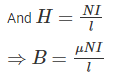Magnetic field density is inversely proportional to length. Hence magnetic flux density increases with decrease in distance.

QUESTION: 20

Ferrites are types of

Solution:

Ferrites are usually ferrimagnetic ceramic compounds derived from iron oxides. Like most of the other ceramics, ferrites are hard, brittle, and poor conductors of electricity.